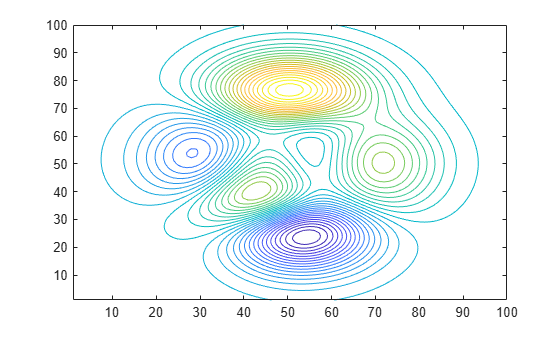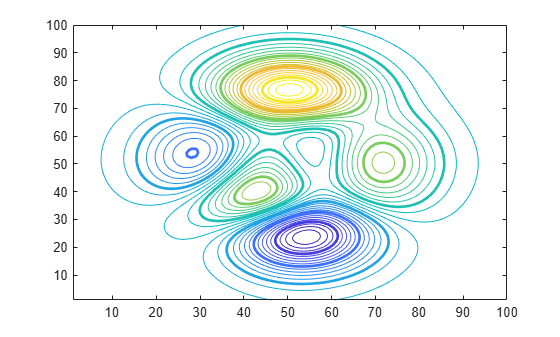# Highlight Specific Contour Levels

This example shows how to highlight contours at particular levels.

Define `Z` as the matrix returned from the `peaks` function.

`Z = peaks(100);`

Round the minimum and maximum data values in `Z` and store these values in `zmin` and `zmax`, respectively. Define `zlevs` as 40 values between `zmin` and `zmax`.

```zmin = floor(min(Z(:))); zmax = ceil(max(Z(:))); zinc = (zmax - zmin) / 40; zlevs = zmin:zinc:zmax;```

Plot the contour lines.

```figure contour(Z,zlevs)```Define `zindex` as a vector of integer values between `zmin` and `zmax` indexed by 2.

`zindex = zmin:2:zmax;`

Retain the previous contour plot. Create a second contour plot and use `zindex` to highlight contour lines at every other integer value. Set the line width to 2.

```hold on contour(Z,zindex,'LineWidth',2) hold off```